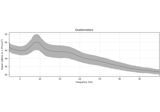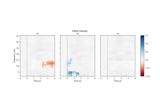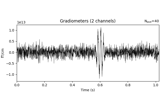# mne.time_frequency.tfr_multitaper#

mne.time_frequency.tfr_multitaper(inst, freqs, n_cycles, time_bandwidth=4.0, use_fft=True, return_itc=True, decim=1, n_jobs=None, picks=None, average=True, verbose=None)[source]#

Compute Time-Frequency Representation (TFR) using DPSS tapers.

Same computation as `tfr_array_multitaper`, but operates on `Epochs` objects instead of `NumPy arrays`.

Parameters:
inst

The epochs or evoked object.

freqs`ndarray`, shape (n_freqs,)

The frequencies in Hz.

n_cycles`float` | `ndarray`, shape (n_freqs,)

The number of cycles globally or for each frequency. The time-window length is thus T = n_cycles / freq.

time_bandwidth`float`, (optional), default 4.0 (n_tapers=3)

Time x (Full) Bandwidth product. Should be >= 2.0. Choose this along with n_cycles to get desired frequency resolution. The number of good tapers (least leakage from far away frequencies) is chosen automatically based on this to floor(time_bandwidth - 1). E.g., With freq = 20 Hz and n_cycles = 10, we get time = 0.5 s. If time_bandwidth = 4., then frequency smoothing is (4 / time) = 8 Hz.

use_fftbool, default `True`

The fft based convolution or not.

return_itcbool, default `True`

Return inter-trial coherence (ITC) as well as averaged (or single-trial) power.

decim`int` | `slice`, default 1

To reduce memory usage, decimation factor after time-frequency decomposition. If `int`, returns tfr[…, ::decim]. If `slice`, returns tfr[…, decim].

Note

Decimation may create aliasing artifacts.

n_jobs

The number of jobs to run in parallel. If `-1`, it is set to the number of CPU cores. Requires the `joblib` package. `None` (default) is a marker for ‘unset’ that will be interpreted as `n_jobs=1` (sequential execution) unless the call is performed under a `joblib.parallel_backend()` context manager that sets another value for `n_jobs`.

picks

Channels to include. Slices and lists of integers will be interpreted as channel indices. In lists, channel type strings (e.g., ```['meg', 'eeg']```) will pick channels of those types, channel name strings (e.g., `['MEG0111', 'MEG2623']` will pick the given channels. Can also be the string values “all” to pick all channels, or “data” to pick data channels. None (default) will pick good data channels. Note that channels in `info['bads']` will be included if their names or indices are explicitly provided.

averagebool, default `True`

If `False` return an `EpochsTFR` containing separate TFRs for each epoch. If `True` return an `AverageTFR` containing the average of all TFRs across epochs.

Note

Using `average=True` is functionally equivalent to using `average=False` followed by `EpochsTFR.average()`, but is more memory efficient.

New in version 0.13.0.

verbose

Control verbosity of the logging output. If `None`, use the default verbosity level. See the logging documentation and `mne.verbose()` for details. Should only be passed as a keyword argument.

Returns:
power

The averaged or single-trial power.

itc

The inter-trial coherence (ITC). Only returned if return_itc is True.

Notes

New in version 0.9.0.

## Examples using `mne.time_frequency.tfr_multitaper`#Frequency and time-frequency sensor analysis

Frequency and time-frequency sensor analysisCompute and visualize ERDS maps

Compute and visualize ERDS mapsTime-frequency on simulated data (Multitaper vs. Morlet vs. Stockwell)

Time-frequency on simulated data (Multitaper vs. Morlet vs. Stockwell)